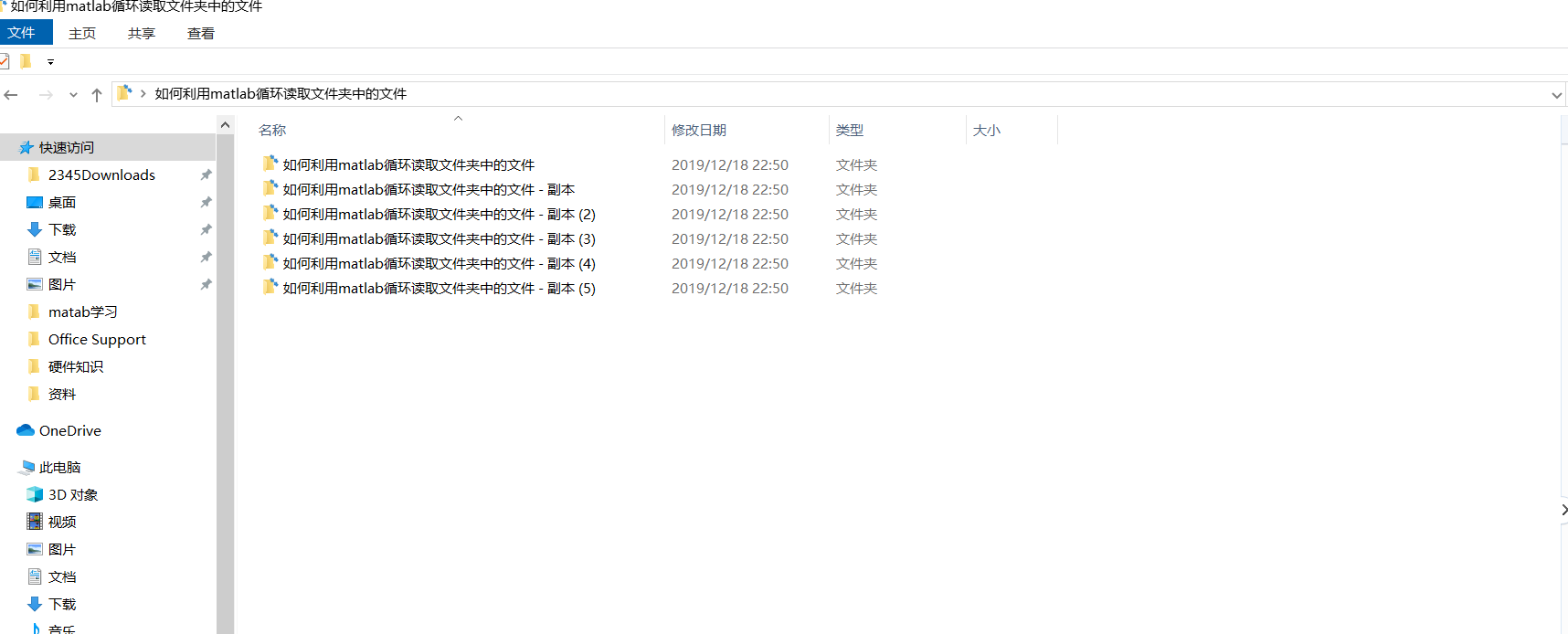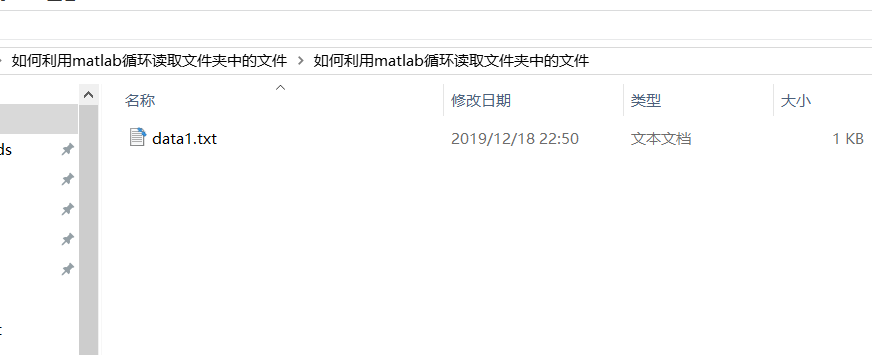• 生成的HEVC的txt文件众多，需要提取需要的bits 手动操作繁杂 针对多文件夹下多个相同的txt文件适用
• MATLAB循环读取excel各行表格代码，操作简单，适合新手学习
• 在桌面创建一个名为《如何利用matlab循环读取文件夹中的文件》的文件夹，在再这个文件夹里面创建几个子文件夹，如下图所示； 第二步：在每个文件夹里面放好数据文件，我这里以.txt文件为列子。 具体的如下图所示...

第一步：创建数据文件夹

在桌面创建一个名为《如何利用matlab循环读取文件夹中的文件》的文件夹，在再这个文件夹里面创建几个子文件夹，如下图所示；第二步：在每个文件夹里面放好数据文件，我这里以.txt文件为列子。

具体的如下图所示：对于上面的文件，为了能当输入文件的顺序时能读取文件中的数据，故编写了如下的代码：

clc
clear
close all;
%% 读取各个文件夹
%———注意修改文件的路径，将《C:\Users\pgl\Desktop\如何利用matlab循环读取文件夹中的文件》修改为自己的文件夹————%
filename1=ls('C:\Users\pgl\Desktop\如何利用matlab循环读取文件夹中的文件');%提取文件名,每次必须将文件名写入。
cd('C:\Users\pgl\Desktop\如何利用matlab循环读取文件夹中的文件')%跳转到要处理的数据所在文件夹
filename=cellstr(filename1);                       %把细胞数组转化为字符串
filename(1:2)=[];                                  %删除filename中的...文件名，不知道哪里来的
num=length(filename);                              %计算文件个数
%% 读取其中一个文件夹中的文件
fileth=1;       %计算第几个文件夹的数据，fileth最大能选择num
%如果选择的文件数大于num，初始化为第一个
if fileth>num
fileth=1;
end
file_location=['C:\Users\pgl\Desktop\如何利用matlab循环读取文件夹中的文件','\',filename(fileth)];
C=cell2mat(file_location);                 %细胞数组转化为字符串
cd(C);                                           %跳转到第fileth个文件夹
all_fileth_filename_char=ls(C);
all_fileth_filename=cellstr(all_fileth_filename_char);                       %把细胞数组转化为字符串
all_fileth_filename(1:2)=[];
num_file=length(all_fileth_filename);%计算文件夹中文件的个数
detail_file_name=1;                          %打算读取的文件序号
%防止因为输入而产生的错误
if detail_file_name>num_file
detail_file_name=1;
end
file_char=all_fileth_filename{detail_file_name};%提取文件的字符

方法二：如何快速读取文件夹里面的同一类型的文件

clc
clear
close all
location='如何利用matlab循环读取文件夹中的文件/';
temp1 = dir([location,  '*.txt']);
cd('如何利用matlab循环读取文件夹中的文件')
N=length(temp1);
for i=1:N
end
%  注意：将需要读取的文件夹放在和程序的同一文件夹内，

展开全文cd 文件夹 文件
• clc;clear; close all cd F:\01_DATA\Data_process\nc... 链接地址：http://blog.sciencenet.cn/blog-3418453-1197697.html 上一篇：[MATLAB]如何将曲线平滑化 下一篇：[MATLAB]方便快捷读取结构体里数个结构体内的数据

clc;clear; close all

cd F:\01_DATA\Data_process\nc

% read filenames of all files in one directory

fileFolder=fullfile('F:\01_DATA\Data_process\nc');

dirOutput=dir(fullfile(fileFolder,'*.nc'));

fileNames={dirOutput.name};

%

for ii = 1:length(fileNames)

filename = fileNames{ii};

end

save dudg_2000_2008.mat

转载本文请联系原作者获取授权，同时请注明本文来自胡振东科学网博客。

链接地址：http://blog.sciencenet.cn/blog-3418453-1197697.html

上一篇：[MATLAB]如何将曲线平滑化

下一篇：[MATLAB]方便快捷读取结构体里数个结构体内的数据

展开全文• matlab循环读取同一文件夹下的多个excel 并整合数据我想用matlab生成一份试excel中，但不知道如何设置单元格宽度和高度，以及边框宽度，字体大小，请各位赐教，谢谢！！！Excel = actxserver('Excel.Application');...

matlab循环读取同一文件夹下的多个excel 并整合数据

我想用matlab生成一份试excel中，但不知道如何设置单元格宽度和高度，以及边框宽度，字体大小，请各位赐教，谢谢！！！

Excel = actxserver('Excel.Application');

set(Excel, 'Visible', 1);

Workbooks = Excel.Workbooks;

Sheets = Excel.ActiveWorkBook.Sheets;

sheet1 = get(Sheets, 'Item', 1);

invoke(sheet1, 'Activate');

Activesheet = Excel.Activesheet;

ActivesheetRange = get(Activesheet,'Range','A1:I1');

set(ActivesheetRange,'MergeCells',1);

set(ActivesheetRange,'HorizontalAlignment',3);

set(ActivesheetRange,'Value','试 卷 分 析');

matlab怎么合并excel单元格并赋值

我想用matlab生成一份试excel中，但不知道如何设置单元格宽度和高度，以及边框宽度，字体大小，请各位赐教，谢谢！！！

Excel = actxserver('Excel.Application');

set(Excel, 'Visible', 1);

Workbooks = Excel.Workbooks;

Sheets = Excel.ActiveWorkBook.Sheets;

sheet1 = get(Sheets, 'Item', 1);

invoke(sheet1, 'Activate');

Activesheet = Excel.Activesheet;

ActivesheetRange = get(Activesheet,'Range','A1:I1');

set(ActivesheetRange,'MergeCells',1);

set(ActivesheetRange,'HorizontalAlignment',3);

set(ActivesheetRange,'Value','试 卷 分 析');

运用matlab合并excel

fn = 'BBB.xls';

S = [s1 s2];

xlswrite(fn,S,'sheet3','A1');

用matlab合并excel表格文件

我想用matlab生成一份试excel中，但不知道如何设置单元格宽度和高度，以及边框宽度，字体大小，请各位赐教，谢谢！！！

Excel = actxserver('Excel.Application');

set(Excel, 'Visible', 1);

Workbooks = Excel.Workbooks;

Sheets = Excel.ActiveWorkBook.Sheets;

sheet1 = get(Sheets, 'Item', 1);

invoke(sheet1, 'Activate');

Activesheet = Excel.Activesheet;

ActivesheetRange = get(Activesheet,'Range','A1:I1');

set(ActivesheetRange,'MergeCells',1);

set(ActivesheetRange,'HorizontalAlignment',3);

set(ActivesheetRange,'Value','试 卷 分 析');

怎样利用matlab去读取一个excel表中多个sheet的数...

我想用matlab生成一份试析到excel中，但不如何设置单宽度和高度，以及边框宽度，还有大小，请各位赐教，谢谢！！！

Excel = actxserver('Excel.Application');

set(Excel, 'Visible', 1);

Workbooks = Excel.Workbooks;

Sheets = Excel.ActiveWorkBook.Sheets;

sheet1 = get(Sheets, 'Item', 1);

invoke(sheet1, 'Activate');

Activesheet = Excel.Activesheet;

ActivesheetRange = get(Activesheet,'Range','A1:I1');

set(ActivesheetRange,'MergeCells',1);

set(ActivesheetRange,'HorizontalAlignment',3);

set(ActivesheetRange,'Value','试 卷 分 析');

展开全文Contents

2.1.fprintf写出数据txt

% 把文件的文件名按一定的规律命名，假如：f001.mat，f002.mat，...

% 在读取的时候则可以使用循环

% f001.mat, f002.mat, f003.mat三个数据文件，其变量名称均为a(1x3的矩阵)

a = [0 0 1];

save f001;

a = [0 0 2];

save f002;

a = [0 0 3];

save f003;

clear;

x = zeros(3); %将三个文件的数据读到 x

for i = 1:3

FileName =

['f00' num2str(i)];

(FileName);

x(i, :) =

end

disp('x = ');

disp(x);

x =

0 0 1

0 0 2

0 0 3

2.1.fprintf写出数据txt

a1 = 1:10;

a2 = 11:20;

fid1 =

fopen('t001.txt','wt'); %若是在txt中,需在w后面加上t,由w变为wt,n才能识别

for i=1:10

fprintf(fid1,'%g n',a1(i));

end

fclose(fid1);

fid2 =

fopen('t002.txt','wt'); %若是在txt中,需在w后面加上t,由w变为wt,n才能识别

for i=1:10

fprintf(fid2,'%g n',a2(i));

end

fclose(fid2);

y = zeros(10,2);

for i = 1:2

FileName =

['t00' num2str(i) '.txt'];

(FileName);

y(:, i) =

a;

end

disp('y = ');

disp(y);

y =

1 11

2 12

3 13

4 14

5 15

6 16

7 17

8 18

9 19

10 20

如果你有所收获，欢迎用微信扫一扫进行打赏，赏金随意。展开全文• MATLAB循环处理一个文件夹内的同种文件并进行处理的方法。 Files = dir(fullfile('D:\Documents\*.mat')); % 读取文件夹内的mat格式的文件 LengthFiles = length(Files); %所有文件的数量 得到Files是一个struct...matlab
• Matlab如何循环读取文件转载http://www.cnblogs.com/woshitianma/p/3724922.html第一种方法①List =dir('*.jpg');第二种方法②I=ones(8,5);第三种方法③ 转载http://www.cnblogs.com/woshitianma/p/3724922.html ...matlab
• 1、matlab批量导入txt文件 2、将txt文件中的英文去除 3、逐行将矩阵输出为excelmatlab txt excel
• 给出了一个多组数据循环读取，并进行特征提取和频谱分析的例子
• matlab循环读取txt文件

千次阅读 2019-01-03 11:38:37
• matlab怎么把计算的数据保存到excel里面？将数据保存到excel文件xlswrite(xlsfile, data, sheet, range); % sheet 和 range可以不指定如:xlswrite('d:\test.xls', data, 'sheet1'); % 将data写入test.xls的工作表...
• 利用strcat函数连接字符串 例如：读取D:\Program\x01~x09 for i = 1:9 Data = load(strcat(‘D:\Program\x0’,num2str(i),’.txt’)); end
• matlab程序读取单片机通过串口传来的IMU角度数据，并用曲线显示出来。上传的值为float型，每个数据后有回车符。范围90~-90主文件 serial_test2.m%%clc;global t;global x;global m;global ii;t = ;m = ;ii = 0...
• 可以通过matlab读取excel表格并画图，可以索引至第n列，功能易实现，可验证
• Matlab如何循环读取文件

千次阅读 2019-09-29 02:03:24
循环读取图片第一种方法①List =dir('*.jpg'); %如需其它图片格式支持，可以自己【重载dir()】函数，实现查找所有图片文件的功能，%如果图片是其它路径，可以用 ["路径" ".扩展名"] 字符串来实现。k =length(dList);...
• matlab读取某个采集生成的振动数据或其他数据，文件夹为当前日期，文件为传的参数.txt，数据为单列数据
• Matlab批量读取数据文件

千次阅读 2020-05-25 15:39:04
文章目录Matlab批量读取数据文件需求缺憾 Matlab批量读取数据文件 初次使用Matlab解决问题，记录一下。 需求 首先说明一下需求，机器能够将18×18的数据矩阵保存到文件中，要做的是读取这个文件，去除首行的无关数据...matlab
• 循环读取文件名，放入元胞数组3. 按文件名循环处理数据，每个文件处理流程如下3.1 根据数据标识去除无效数据 3.2 读取待处理数据，存入二维数组3.3 数据运算，绘图3.4 新建文件夹dat_image，以文件名变量命名另存...
• % n 为数据文件个数 % 数据文件格式为： mat dat txt xls % data 为输入数据的结构体  % 取出任何一个来用的语句为 data{i} i表示第i次读入文件！（如果进行了顺序组合，最后只剩下data{1}！） % 例如...
• 现在有若干excel文件，每个文件只有一列且无标题，文件名分别为aa_1.xlsx,aa_2xlsx,aa_3xlsx等等，以此类推，在matlab中如何利用for循环读取数据，在工作区中每一个表分别对应一个文件中的数据，比如工作区中a1对应...matlab 有问必答MATLAB
• 使用MATLAB的GUI界面操作，实时读取麦克风，并绘制时域图和频域图。主要是使用循环实现连续读取，在循环体内进行快速傅里叶变换（fft），得到频率对应的能量。
• HDF是一种广泛用于科学数据共享的国际标准数据格式，正确高效地读取HDF文件数据是对其进行应用的基础。本文首先介绍了HDF文件格式，以及几种常用的HDF文件读取...最后，本文给出了利用MATLAB读取NCEP数据的应用实例。
• 有时候我们需要对某个文件夹或者多个文件夹中的Excel文件进行读取，然后处理。那么怎么实现呢？ 以一个文件夹下的Excel文件进行读取为例。 文件地址：E:\Study of Lab\Coding\Experience DatePhoto\Excel 对文件...matlab excel
• matlab hdf 读取

2021-04-18 15:38:31
大量数据读取问题:可以考虑使用循环分批读取 (特别是在各数据是独立的时候) , 或者使用稀疏矩阵来实现。 另外, 也可参考《深入浅出 MATLAB 7_X 混合编程》一......MATLAB支持五种图像类型,即索引图像、灰度图像、...
• 使用Matlab编写的小程序，用于批量读取Excel内容。本程序中用于读取特定字符的内容，可自行根据需要更改...

matlab循环读取数据matlab 订阅